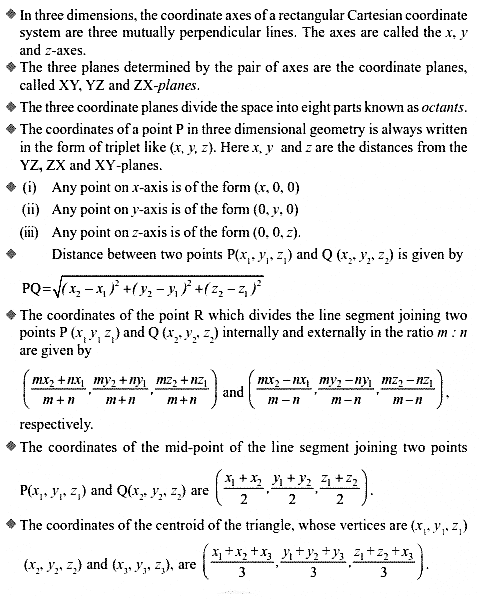# NCERT Solutions Class 11 Maths Chapter 12 Introduction To 3D Geometry

Ncert Solutions for class 11 subject Maths Chapter 12 Introduction To 3D Geometryin pdf Best Free NCERT Solutions for class 1 to 12 in pdf NCERT Solutions, cbse board, Maths, ncert Solutions for Class 11 Maths, class 11 Maths ncert solutions, Introduction To 3D Geometry, Class 11, ncert solutions chapter 12 Introduction To 3D Geometry, class 11 Maths, class 11 Maths ncert solutions, Maths ncert solutions class 11, Ncert Solutions Class 11 Mathematics Chapter 12 Introduction To 3D Geometry

## Formulas and definitions Chapter 12 Class 11 Maths Introduction To 3D Geometry Ncert Solutions## Exercise Solutions 12.1 Chapter 12 Class 11 Maths Introduction To 3D Geometry Ncert Solutions

EXERCISE 12.1 1. A point is on the x-axis. What are its y-coordinate and z-coordinates? 2. A point is in the XZ-plane. What can you say about its y-coordinate? 3. Name the octants in which the following points lie: (1,. 2, 3), (4, –2, 3), (4, –2, –5), (4, 2, –5), (– 4, 2, –5), (– 4, 2, 5), (–3, –1, 6) (2, – 4, –7). 4. Fill in the blanks: (i) The x-axis and y-axis taken together determine a plane known as_______. (ii) The coordinates of points in the XY-plane are of the form _______. (ii

## Exercise Solutions 12.2 Chapter 12 Class 11 Maths Introduction To 3D Geometry Ncert Solutions

1. Find the distance between the following pairs of points: (i) (2, 3, 5) and (4, 3, 1) (ii) (–3, 7, 2) and (2, 4, –1) (iii) (–1, 3, – 4) and (1, –3, 4) (iv) (2, –1, 3) and (–2, 1, 3).
2. Show that the points (–2, 3, 5), (1, 2, 3) and (7, 0, –1) are collinear.
3. Verify the following: (i) (0, 7, –10), (1, 6, – 6) and (4, 9, – 6) are the vertices of an isosceles triangle. (ii) (0, 7, 10), (–1, 6, 6) and (– 4, 9, 6) are the vertices of a right angled triangle. (iii) (–1, 2, 1), (1,

4. Find the equation of the set of points which are equidistant from the points (1, 2, 3) and (3, 2, –1).

5. Find the equation of the set of points P, the sum of whose distances from A (4, 0, 0) and B (– 4, 0, 0) is equal to 10.

## Exercise Solutions 12.3 Chapter 12 Class 11 Maths Introduction To 3D Geometry Ncert Solutions

1. Find the coordinates of the point which divides the line segment joining the points (– 2, 3, 5) and (1, – 4, 6) in the ratio (i) 2 : 3 internally, (ii) 2 : 3 externally.

2. Given that P (3, 2, – 4), Q (5, 4, – 6) and R (9, 8, –10) are collinear. Find the ratio in which Q divides PR.

3. Find the ratio in which the YZ-plane divides the line segment formed by joining the points (–2, 4, 7) and (3, –5, 8).

4. Using section formula, show that the points A (2, –3, 4), B (–1, 2, 1) and (0, 1/3 , 2) are collinear.

5. Find the coordinates of the points which trisect the line segment joining the points P (4, 2, – 6) and Q (10, –16, 6).

## Exercise Miscellaneous Chapter 12 Class 11 Maths Introduction To 3D Geometry Ncert Solutions

1. Three vertices of a parallelogram ABCD are A(3, – 1, 2), B (1, 2, – 4) and C (– 1, 1, 2). Find the coordinates of the fourth vertex.

2. Find the lengths of the medians of the triangle with vertices A (0, 0, 6), B (0,4, 0) and (6, 0, 0).

3. If the origin is the centroid of the triangle PQR with vertices P (2a, 2, 6), Q (– 4, 3b, –10) and R(8, 14, 2c), then find the values of a, b and c.

4. Find the coordinates of a point on y-axis which are at a distance of 5 root 2 from the point P (3, –2, 5).

5. A point R with x-coordinate 4 lies on the line segment joining the points P(2, –3, 4) and Q (8, 0, 10). Find the coordinates of the point R.

6.If A and B be the points (3, 4, 5) and (–1, 3, –7), respectively, find the equation of the set of points P such that ...

### NCERT Books Free Pdf Download for Class 5, 6, 7, 8, 9, 10 , 11, 12 Hindi and English Medium

 Mathematics Biology Psychology Chemistry English Economics Sociology Hindi Business Studies Geography Science Political Science Statistics Physics Accountancy